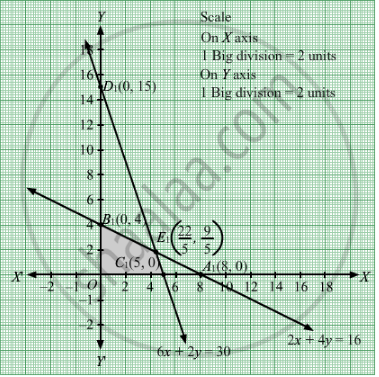# A Manufacturer of Furniture Makes Two Products : Chairs and Tables. Processing of These Products is Done on Two Machines a and B. - Mathematics

Sum

A manufacturer of Furniture makes two products : chairs and tables. processing of these products is done on two machines A and B. A chair requires 2 hrs on machine A and 6 hrs on machine B. A table requires 4 hrs on machine A and 2 hrs on machine B. There are 16 hrs of time per day available on machine A and 30 hrs on machine B. Profit gained by the manufacturer from a chair and a table is Rs 3 and Rs 5 respectively. Find with the help of graph what should be the daily production of each of the two products so as to maximize his profit.

#### Solution

Let  chairs and y tables were produced.
Number of chairs and tables cannot be negative.
Therefore, $x, y \geq 0$

The given information can be tabulated as follows:

 Time on machine A(hrs) Time on machine B (hrs) Chairs 2 6 Tables 4 2 Availability 16 30

Therefore, the constraints are

$2x + 4y \leq 16$
$6x + 2y \leq 30$

Profit gained by the manufacturer from a chair and a table is Rs 3 and Rs 5 respectively. Therefore, profit gained from x chairs and y tables is Rs 3x and Rs 5y.
Total profit = Z =  $3x + 5y$  which is to be maximised

Thus, the mathematical formulat​ion of the given linear programmimg problem is

Max Z =  $3x + 5y$
subject to

$2x + 4y \leq 16$
$6x + 2y \leq 30$

$x, y \geq 0$

First we will convert inequations into equations as follows:
2x + 4y = 16, 6x + 2y =30, x = 0 and y = 0
Region represented by 2x + 4y ≤ 16:
The line 2x + 4y = 16 meets the coordinate axes at A1(8, 0) and B1(0, 4) respectively. By joining these points we obtain the line 2x + 4y = 16. Clearly (0,0) satisfies the 2x + 4y = 16. So, the region which contains the origin represents the solution set of the inequation 2x + 4y ≤ 16.
Region represented by 6x + 2y ≤ 30:
The line 6x + 2y =30 meets the coordinate axes at C1(5, 0) and D1(0, 15) respectively. By joining these points we obtain the line 6x + 2y =30 . Clearly (0,0) satisfies the inequation 6x + 2y ≤ 30. So,the region which contains the origin represents the solution set of the inequation 6x + 2y ≤ 30.
Region represented by x ≥ 0 and y ≥ 0:
Since, every point in the first quadrant satisfies these inequations. So, the first quadrant is the region represented by the inequations x ≥ 0, and ≥ 0.
The feasible region determined by the system of constraints 2x + 4y ≤ 16, 6x + 2y ≤ 30, x ≥ 0, and y ≥ 0 are as follows.The corner points are O(0, 0), B1(0, 4), E1

$\left( \frac{22}{5}, \frac{9}{5} \right)$ and C1(5, 0).

The values of Z at these corner points are as follows
 Corner point Z= 3x + 5y O 0 B1 20 E1 22.2 C1 15

The maximum value of Z is 22.2 which is attained at B1
$\left( \frac{22}{5}, \frac{9}{5} \right)$ Thus, the maximum profit  is of Rs 22.20 obtained when $\frac{22}{5}$ units of chairs  and $\frac{9}{5}$  units of tables are produced.

Concept: Graphical Method of Solving Linear Programming Problems
Is there an error in this question or solution?

#### APPEARS IN

RD Sharma Class 12 Maths
Chapter 30 Linear programming
Exercise 30.4 | Q 10 | Page 51